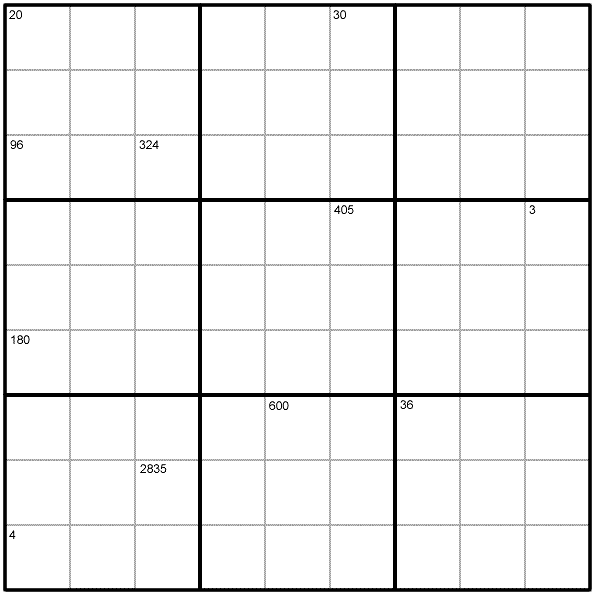#### You may also likeMake a set of numbers that use all the digits from 1 to 9, once and once only. Add them up. The result is divisible by 9. Add each of the digits in the new number. What is their sum? Now try some other possibilities for yourself!### N000ughty Thoughts

How many noughts are at the end of these giant numbers?### DOTS Division

Take any pair of two digit numbers x=ab and y=cd where, without loss of generality, ab > cd . Form two 4 digit numbers r=abcd and s=cdab and calculate: {r^2 - s^2} /{x^2 - y^2}.

# Product Sudoku

##### By Henry Kwok### Rules of Product Sudoku

Like a conventional Sudoku, this Product Sudoku has two basic rules:
1. Each column, row and $3 \times 3$ subgrid must have the numbers 1 to 9.
2. No column, row or subgrid can have two cells with the same number.

The puzzle can be solved with the help of the numbers in the top parts of certain cells. These numbers are the products of the digits in all the cells horizontally and vertically adjacent to the cell.

### A Short Demonstration

The cell in the top left corner of this Sudoku contains the number 20. 20 is the product of the digits in the two adjacent cells, which therefore must contain the digits 4 and 5. The 5 cannot go in the cell below the top left hand corner because 5 is not a factor of 96 (the product shown in the third cell down on the left hand side of the puzzle). Therefore 5 must be entered into the cell to the right of the cell containing 20 and 4 in the cell below.

A printable version of the problem can be found here.